cancel
Showing results for
Did you mean:## How to put the row and column subtotals in front of the matrix

Scenario:

Although there is no direct operation to put the total row in front of the matrix column in Power bi, we can still make it by using below two methods.

1. Use the summarized function to form a new table of data string “Total”, and add a space string at the top of it, then add the new table to the original one through the union function, and finally, “Total with spaces” will go to the top automatically when they match alphabetically.

2. Create a special column that contains “Total” and " Total" contains empty characters. According to the alphabetical order, it will be the first column in the matrix. Create a measure to determine whether the selected is " Total", Yes, SUM is performed, if not, the current value is assigned.

Sample Data:

‘Row Table’: Here's the sample table that contains the data between 2020 / 1 / 1 and 2020 / 1 / 3 to calculate the row subtotal.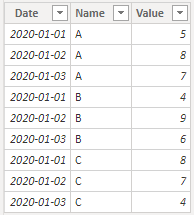‘Column Table’: Here's the sample table that contains the data between 2020 / 1 / 1 and 2020 / 1 / 3 to calculate the column subtotal.Expected output:

‘Row Table’: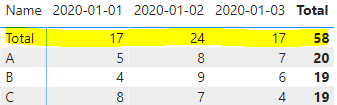‘Column Table’: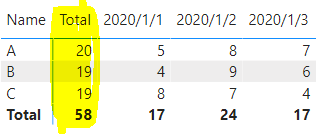Guideline:

There are two methods:

• Method 1:
Row Subtotal：
1. Create a table of calculations grouped by Date add two columns named Name and Value, where ‘Name’ is the “Total’ string with spaces and value is that in the original ‘row Table” to be added as per the Date group.
2. As far as we can see, I added three rows to the end of the original data table and used the name column as " Total", created a matrix and placed it, rows = ‘Row Table’[name], column = ‘Row Table’[name], values = ‘Row Table’[Value].

Column Subtotal：

1. Create a table of calculations grouped by name, add two columns named Date and Value, where ‘Date’ is the “Total’ string with spaces and value is that in the original ‘column Table” to be added as per the name group.

2. As far as we can see, I added three rows to the end of the original data table and used the name column as " Total", created a matrix and placed it, rows = ‘Column Table’[name], column = ‘Column Table’[name], values = ‘Column Table’[Value].

• Method 2:
Row Subtotal：
1. Use distinct to de-duplicate the data of 'Row Table'[Name] to form a Table1, and use the datatable() function to form a Name column. The data is Table2 with a space string " Total", and finally, use Union to append the two tables to form a separate table ‘Name select’, and create the relationship between the table ‘Name select’ and the table ‘Row Table’.
2. Put the data in the table into the matrix in this form (Row= 'Column Table'[Name], Column= 'Date select’[Date], Values= 'Column Table'[Value]), then you will see “Total” in the matrix, since  the string with a space is sorted alphabetically, it will be sorted to the left automatically. Then we created a measure to determine whether the selection is " Total", Yes, SUM will be performed, if not, the current value will be assigned.

Column Subtotal：

1. Use distinct to de-duplicate the data of 'Column Table'[Date] to form a Table1, and use the datatable() function to form a date column. The data is Table2 with a space string" Total", and finally, use Union to append the two tables to form a separate table ‘Date select’, and create the relationship between the table ‘Date select’ and the table ‘Column Table’.

2. Put the data in the table into the matrix in this form (Row= 'Column Table'[Name], Column= ‘Date select’[Date], Values= ‘Column Table’[Value]), then you will see “Total” in the matrix, since  the string with a space is sorted alphabetically, it will be sorted to the left automatically. Then we create a measure to determine whether the selection is " Total", Yes, SUM will be performed, if not, the current value will be assigned.

Operations:

• Method 1:

Row Subtotal：

1. Create a calculated table to group by Date, add two columns named Name and Value, Name is the string" Total" with spaces, and Value is the value in the original table ‘Row Table’ to be added according to the Date group.

``````‘Row SubTotal’ =
VAR _summarize =
SUMMARIZE (
'‘Row Table’',
[Date],
"Name", " Total",
"Value", SUM ( ’Row Table’[Value] )
)
RETURN
UNION ( '‘Row Table’', _summarize )``````

Note：

The space character in " Total" will automatically rank Total according to the alphabetical order to the leftmost side of the matrix to prevent confusion due to the alphabetical order.

Result：Step analysis:

Use the summarize function to create a calculated table with the Date column as the group, the Name column as the “Total”, and the Value column data as the added calculated column table after grouping, and then use the union function to append the calculated table and ‘Row Table’.

1. As far as we can see, I added three rows to the end of the original data table and used the name column as " Total", creating a matrix and placing it, rows = ‘Row Table’[name], column = ‘Row Table’[name], values = ‘Row Table’[Value].

Result: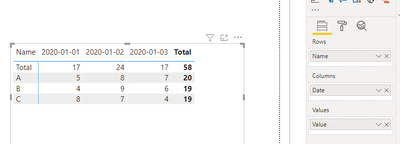Step analysis:

The Name column of the new formed table “Row Sub Total” is composed of ABC type data and " Total". After placing it in the matrix, " Total" will be placed on the leftmost side according to the alphabetical order due to the space string.

Column Subtotal

1. Create a table of calculations grouped by name, add two columns named Date and Value, where ‘Date’ is the “Total’ string with spaces and value is that in the original ‘column Table” to be added as per the name group.

``````‘Column Sub Total’ =
VAR _summarize =
SUMMARIZE (
'‘Column Table’',
[Name],
"Date", " Total",
"Value", SUM ( ’Column Table’[Value] )
)
RETURN
UNION ( '‘Column Table’', _summarize )``````

Result：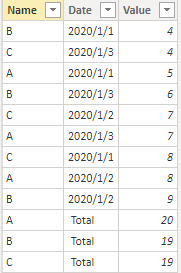Step analysis:

Use the summarize function to create a calculated table with the Name column as the grouping and the Date column as " Total", and the Value column data as the added calculated column table after grouping, and then use the union function to append the calculated table and ‘Column Table’.

1. As far as we can see, I added three rows to the end of the original data table and used the name column as " Total", created a matrix and placed it, rows = ‘Column Table’[name], column = ‘Column Table’[name], values = ‘Column Table’[Value].

Result：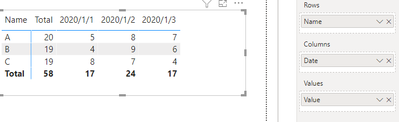Step analysis:

The Date column of the new table ‘Column Sub Total’ formed is composed of 2020/1/1 date type data and " Total". After placing it in the matrix, " Total" will be placed on the leftmost side according to the alphabetical order due to the space string.

• Method 2:

Row Subtotal:

1. Use distinct to de-duplicate the data of ‘Row Table’[Name] to form a Table1, and use the datatable() function to form a Name column. The data is Table2 with a space string " Total", and finally, use Union to append the two tables to form a separate table ‘Name select’, and create the relationship between the table ‘Name select’ and the table ‘Row Table’.

``````‘Name select’ =
UNION (
DISTINCT ( ’Row Table’[Name] ),
DATATABLE ( "Name", STRING, { { " Total" } } )
)   ``````

Result: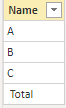Step analysis:

Use distinct to de-duplicate the name in the table ‘Row Table’, use datatable() to create data containing " Total" in the Name column, and perform union to form ABC type data and " Total" table ‘Name select’.

1. Put the data in the table into the matrix in this form (Row= 'Column Table'[Name], Column= 'Date select’[Date], Values= 'Column Table'[Value]), then you will see “Total” in the matrix, since  the string with a space is sorted alphabetically, it will be sorted to the left automatically. Then we created a measure to determine whether the selection is " Total", Yes, SUM will be performed, if not, the current value will be assigned.

``````Row Subtotal second =
IF (
SELECTEDVALUE ( '‘Name select’'[Name] ) = " Total",
CALCULATE ( SUM ( ’Row Table’[Value] ), ALL ( '‘Name select’'[Name] ) ),
SUM ( ’Row Table’[Value] )
)``````

Result: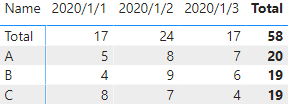Step analysis:

When ‘Name select’ '[Name] Selected data " Total" when the value of the Value will be summed according to Date, otherwise, it returns the current value of.

Column Subtotal：

1. Use distinct to de-duplicate the data of ‘Column Table’[Date] to form a Table1, and use the datatable() function to form a date column. The data is Table2 with a space string " Total", and finally, use Union to append the two tables to form a separate table ‘Date select’, and create the relationship between the table ‘Date select’ and the table.

``````‘Date select’ =
UNION (
DISTINCT ( ’Column Table’[Date] ),
DATATABLE ( "Date", STRING, { { " Total" } } )
)``````

ResultStep analysis:

Use distinct to de-duplicate the name in the table ‘Column Table’, use datatable() to create data containing " Total" in the date column, and perform union to form 2020/1/1 date type data and" Total" table ‘Date select’.

1. Put the data in the table into the matrix in this form (Row= 'Column Table'[Name], Column= ‘Date select’[Date], Values= ‘Column Table’[Value]), then you will see “Total” in the matrix, since the string with a space is sorted alphabetically, it will be sorted to the left automatically. Then we created a measure to determine whether the selection is " Total", Yes, SUM will be performed, if not, the current value will be assigned.

``````Column Subtotal second =
IF (
SELECTEDVALUE ( ’Date select’'[Date] ) = " Total",
CALCULATE ( SUM ( ’Column Table’[Value] ), ALL ( ’Date select’'[Date] ) ),
SUM ( ’Column Table’[Value] )
)``````

Result：Step analysis:

When ‘Date select’'[Date] Selected data " Total" when the value of the Value will be summed according to name, otherwise, it returns the current value of.

Output:

This is the expected table chart.

The blank character before the " Total" makes it the first column in the Matrix because the columns in the Matrix of Power BI are sorted in ascending order from the first character.

We can verify if the results are correct by adding the data.
Add the following data to the two original tables:

Row Subtotal:Column Subtotal:The results of the matrix are as follows:The result is the same as I expected.

Please refer to the attachment below for details.

Author:  Yang Liu

Reviewer: Ula Huang, Kerry Wang